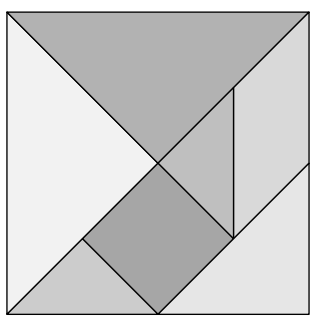시간 제한 메모리 제한 제출 정답 맞은 사람 정답 비율
8 초 (추가 시간 없음) 512 MB 0 0 0 0.000%

## 문제

Chen, your pretty sister, got absorbed in a tiling puzzle called the tangram. Suddenly, she rushed to you and asked for your help, saying "Please, please help me solve this problem! I've been stuck on this for an hour, but I can't figure out how to solve it!" Your pretty, cute, lovely sister is asking for your help, so you have to resolve the problem for her immediately, but... can you really do that?

The tangram is a puzzle considered to be rooted in China. It involves seven pieces, each of which has its specific shape. The objective of this puzzle is to form a given shape using all seven pieces. Each piece can be placed anywhere, and rotated and flipped in any way, but cannot overlap with another one.

The seven pieces consist of the following (see figure):

• Two small right triangles (the lengths of the edges are 25√2, 25√2 and 50),
• One medium right triangle (the lengths of the edges are 50, 50 and 50√2),
• Two big right triangles (the lengths of the edges are 50√2, 50√ 2 and 100),
• One square (the length of the side is 25√2), and
• One parallelogram (the lengths of the sides are 25√2 and 50).Given the shape of a polygon P, your program should determine whether the shape can be made by the seven pieces. To make the problem simpler, you may assume the following:

• Each edge of P is parallel to one of the x-axis, the y-axis, y = x or y = -x.
• P is connected, and has no hole in it. Furthermore, P is a simple polygon, i.e. it has neither self-crossing edges nor self-touching edges.

## 입력

The input begins with a line that contains a single integer N (3 ≤ N ≤ 30), which indicates the number of the vertices in P. The next N lines denote the coordinates of the vertices of P in clockwise order. Each line contains exactly four non-negative integers abc and d, separated by a space. It represents that the corresponding vertex is at (a + b√2, c + d√2). No coordinate exceeds 1000.

## 출력

Print "Yes" in a line if the given shape is solvable, or "No" otherwise.

## 예제 입력 1

4
0 0 0 0
0 0 100 0
100 0 100 0
100 0 0 0


## 예제 출력 1

Yes


## 예제 입력 2

4
0 50 0 0
0 0 0 50
0 50 0 100
0 100 0 50


## 예제 출력 2

Yes